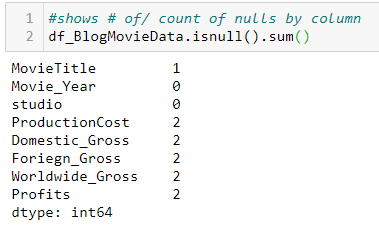# Becoming a Data Alchemist — Dataframes

## Introduction

In my previous post I covered my background and goals for Data Science Bootcamp… Becoming a Data Alchemist (see post). Now I am going to introduce you to Python and one of its core tools for working with data, the Dataframe. Having been schooled in C.P.G. Marketing in Corporate America, I have gained a certain degree of bias for Excel and performing analysis the “Excel way”. However, in bootcamp, it’s the “Python way” or the highway! As I am learning this new environment, I am finding myself constantly comparing the keystrokes required to perform certain tasks in Excel vs. the same and or similar tasks in Python. As I continue to gain proficiency, I am beginning to see the power of Python. Certainly, the learning curve for Python is higher than that of Excel, but as I am discovering the effort put forth in learning the “Python way” is paying off in creative flexibility. Before I get “too fancy” with my tutorials I am going to start with the basics. As we continue our journey in becoming data alchemists, I will work to ramp up the sophistication of my spells 😊.

In this three-part tutorial, this being part-one, I will introduce you to the Dataframe, a central tool in Python, and what I would consider Pythons version of the Excel Worksheet. I have developed this tutorial as the precursor to part two and three in manner which get progressively more technical. Here I introduce you to the “basic” coding required to get to know and clean data using Dataframes. In part two of this tutorial I introduce more “advanced” techniques for summarizing and formatting analysis with Dataframes and in part three I cover some tips on formatting data using multiple formats in one column. Now lets get started with this tutorial!

What is a DataFrame?

Like the Excel worksheet, the Dataframe is used to view, clean, and transform data into insights. Essentially think rows and columns, pivot tables, functions etc, all of the functionality you would expect in a worksheet is mostly available in Dataframes. However, unlike Excel worksheets, Dataframes are not visible until you load them with data and display them. Below, I will cover this simple step within my first example, but before showing you that code, I want to give you a preview of the tasks I will be covering in this tutorial. I selected some basics transformations that frequently need to be available prior to performing analysis.

Common Data Preparation Tasks

`# Code snippets shown in grey for easy cutting and pasting`
` import pandas as pd`

`# read file stored in current working directory, using excel file here`
` df_BlogMovieData = pd.read_excel(‘BlogMovieData.xlsx’) df_BlogMovieData`

Step 2. Cleaning Up Your Data — Finding Nulls, Understanding Data Types, Changing Data Types, and Formatting Data

• Checking for Nulls in our columns:
`df_BlogMovieData.isnull().sum()`Count the nulls for each column
• Filling Blanks or Nulls columns with 0
`# Fill nulls with 0df_BlogMovieData.fillna(0, inplace=True)`
• Changing data types if required, and format your numbers to improve comprehension
`df_BlogMovieData[“Profits”].astype(float) #(float, int,other)df_BlogMovieData.head().style.format({‘ProductionCost’: ‘\${:,.0f}’, ‘Domestic_Gross’ : ‘\${:,.0f}’,’Foriegn_Gross’ : ‘\${:,.0f}’, ‘Worldwide_Gross’ : ‘\${:,.0f}’,’Profits’ : ‘\${:,.0f}’})`

Step 3. Making Changes to the Structure of your Dataframes — Delete Columns, Rename Columns, Combining Columns

• Get Column Names
`df_BlogMovieData.columns`
• Drop Column Names
`df_BlogMovieData.drop(‘Profits’, axis=1, inplace = True)`
• Rename Columns
`df_BlogMovieData.rename(columns={“studio”: “Movie_Studio”}, inplace = True)`
• Add Columns & Combine Numbers
`df_BlogMovieData[‘TotalProfits’] =(df_BlogMovieData[‘Worldwide_Gross’] — df_BlogMovieData[‘ProductionCost’])`
• Summing Column
`TheTotalProfits = df_BlogMovieData[‘TotalProfits’].sum()`
• Sorting Column
`TheTotalProfits = df_BlogMovieData[‘TotalProfits’].sum()`

Step 4. Getting More Advanced — Extracting Data from Dataframe Columns using .apply(Lambda with .split())

Now that you have a sense of the basics, lets look at something a little more advanced but necessary when cleaning data… extracting substrings from larger strings. In python there are several ways this can be done. Below I have shown an intuitive way for the beginner to perform string extraction. In Excel we use — “Left”, “Right” or “Mid”. In Python its not quite that simple. In Python we use a combination of functions “.split()”, “apply()” and something called “Lambda” to access each element in your Dataframe. See the below example showing extracting left, mid, and right using hypothetical characters”~” & “#” for this example.

• Sorting Column
`TheTotalProfits = df_BlogMovieData[‘TotalProfits’].sum()`
• Get left
`GetTheLeft = df_BlogMovieData[“MovieTitle”].apply(lambda x: x.split(‘~’) if x.find(“~”) != -1 else None)`# Essentially . apply() passes in our element, cell by cell, in the lambda we look for “~”, if it finds it, it splits the string at the “~” and returns a list [0,1] in two parts at locations 0, and 1. The x.split is the first location and returns the string on the left side and passed into our variable.
• Get mid
`GetTheMiddle = df_BlogMovieData[“MovieTitle”].apply(lambda x: x.split(‘~’).split(‘#’) if x.find(“~”) != -1 else None)`# similar to above, except here we find if a “~” exists in our string, if so split at “#”, which returns two strings. We access the first string using x.split and the split it again at the “~” but this time instead of grabbing the first location() we grab the second with x.split which is then inserted into our variable.
• Get right
`GetTheRight = df_BlogMovieData[“MovieTitle”].apply(lambda x: x.split(‘#’) if x.find(“~”) != -1 else None)`# Similar to left above, except change the character in which we split and access the second position vs. first position in returned variable ().
`print (GetTheLeft)`
`print (GetTheMiddle)print (GetTheRight)`

Summary & Final Note

Above I covered some of the basics of Dataframes. My aim here and beyond is to provide a gentle introduction to Python in a manner that novices can understand. In future blogs we will get more advanced with material (See (Summarizing data in DataFrames, and Formatting Frustrations with df.describe()). I look forward to seeing you in our next adventure in becoming Data Alchemists!

Next Stop — DATA ALCHEMY!

--

--